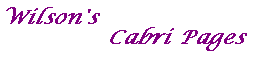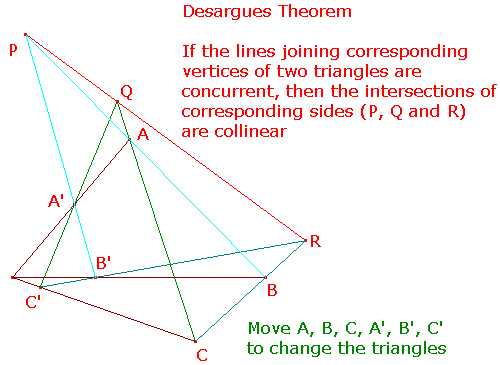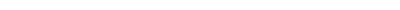The dual of Desargues Theorem states that If the intersections of corresponding sides of two triangles are collinear, then the lines joining corresponding vertices are concurrent. This is the converse of Desargues Theorem!Download desargues.figMain Cabri PageClassical Theorems